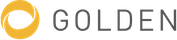# Doping (semiconductor)

Introduction of specific impurities to a semiconductor for the purpose of modulating it’s electrical, optical and structural properties.

### All edits

Edits on 28 Apr, 2020
Infobox (+1 properties)
Infobox
Wikidata entity ID
Q1130571
Edits on 17 Jan, 2020
Article
Article

For intrinsic semiconductors or undoped semiconductors the carrier concentration, electron concentration, and hole concentration are all equivalent. Any charge carriers present in the lattice are due to electrons being thermally excited to the conduction band leaving behind an equal number of holes in the valence band.

...

Introducing dopant atoms into the semiconductor lattice increases the carrier concentration improving the conductivity of the material. Using a group V element as the dopant increases the number of electrons present due to these elements having an extra valence electron. This produces an n-type semiconductor where the charge carriers for conduction are electrons. Introducing group III elements to the lattice increases the number of holes producing a p-type semiconductor where the holes are the charge carriers.

Description (+136/-20 characters)
Article (+1289 characters)

### Doping (semiconductor)

Semiconductor doping

Introduction of specific impurities to a semiconductor for the purpose of modulating it’s electrical, optical and structural properties.

Article

Doping a semiconductor influences it’s electrical, optical and structural properties.

For intrinsic semiconductors or undoped semiconductors the carrier concentration, electron concentration, and hole concentration are all equivalent. Any charge carriers present in the lattice are due to electrons being thermally excited to the conduction band leaving behind an equal number of holes in the valence band.

Introducing dopant atoms into the semiconductor lattice increases the carrier concentration improving the conductivity of the material. Using a group V element as the dopant increases the number of electrons present due to these elements having an extra valence electron. This produces an n-type semiconductor where the charge carriers for conduction are electrons. Introducing group III elements to the lattice increases the number of holes producing a p-type semiconductor where the holes are the charge carriers.

Combining semiconductors with different dopants and concentrations of dopant is the basis for many electrical components such as:

• Bipolar junction transistors
• Field-effect transistors
• Diodes
• Light-emitting diodes
• Lasers
• Solar Cells
• Photodetectors

Semiconductor doping can be performed several ways, examples include:

• Ion implantation
• Diffusion
• Vapor-phase epitaxy
Edits on 1 Jan, 2017